# How To Add Equations To PowerPoint Quickly and Easily

Mar 10, 2023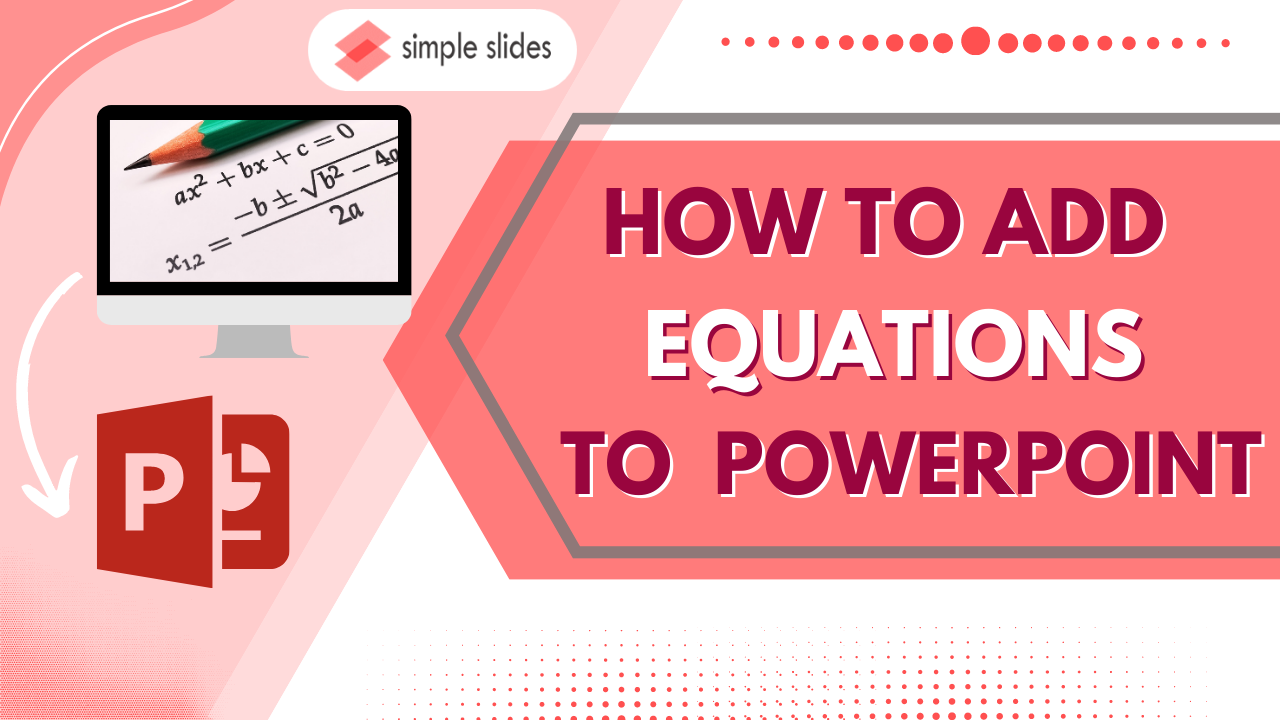Do you need to learn to insert mathematical equations into a PowerPoint presentation?

• How to manually add a new equation.

• How to customize a Microsoft equation.

• Frequently asked questions about how to insert equations in PowerPoint presentations.## How to Insert Equations in Your PowerPoint Presentation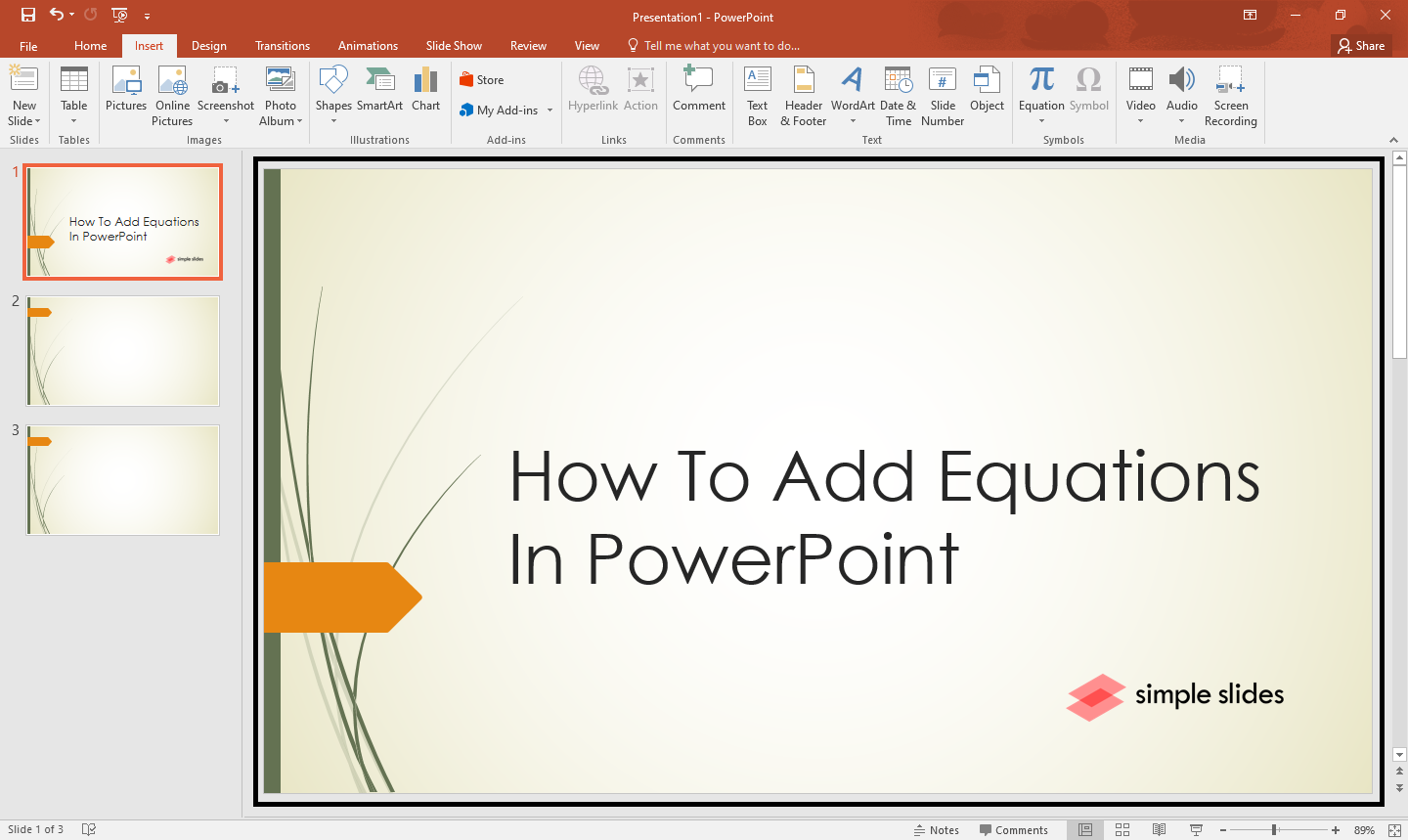2. Select a specific slide in PowerPoint, then select the "Insert" tab, and in the "Symbols group," click "Equation."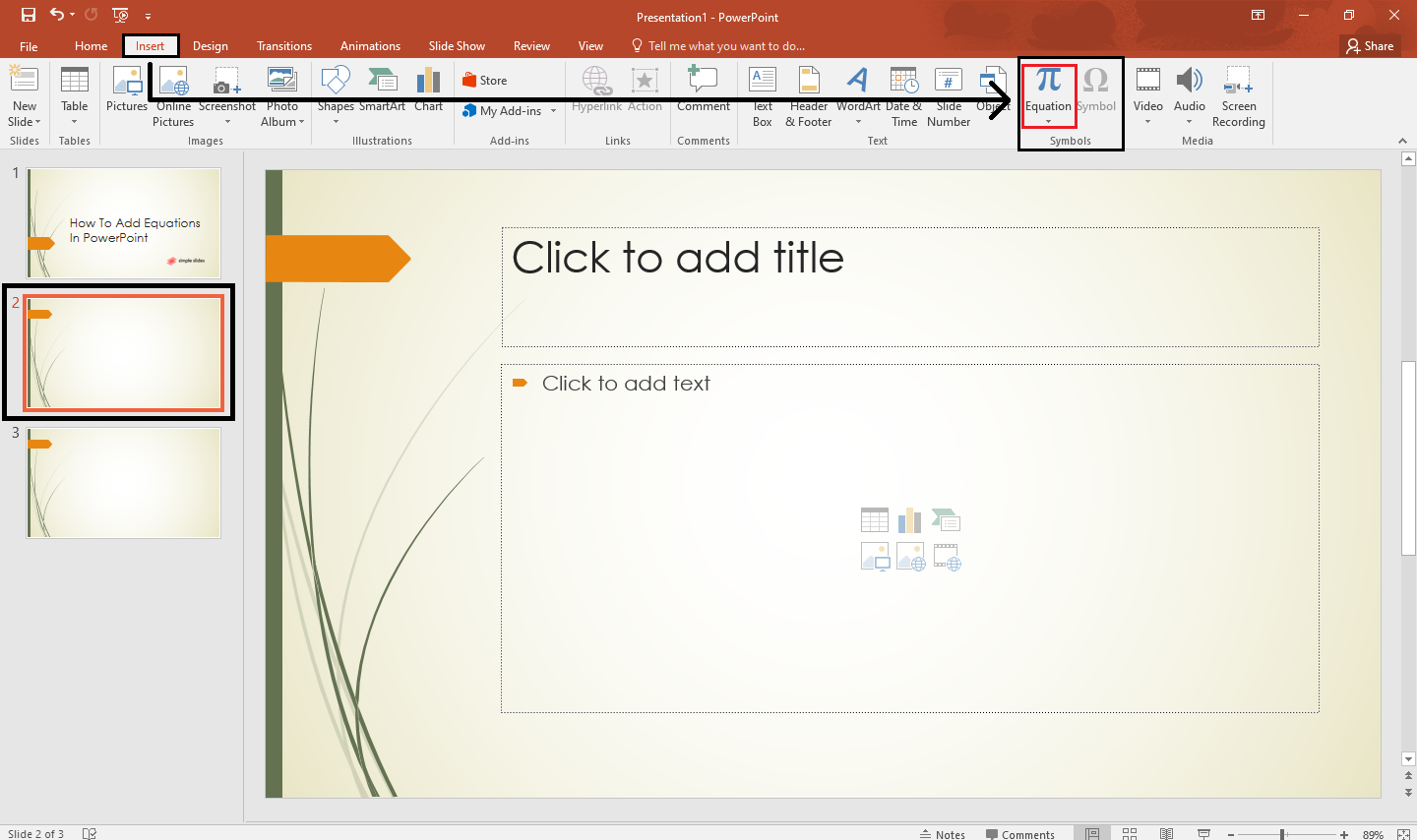3. In the drop-down list, click a specific option for your equation.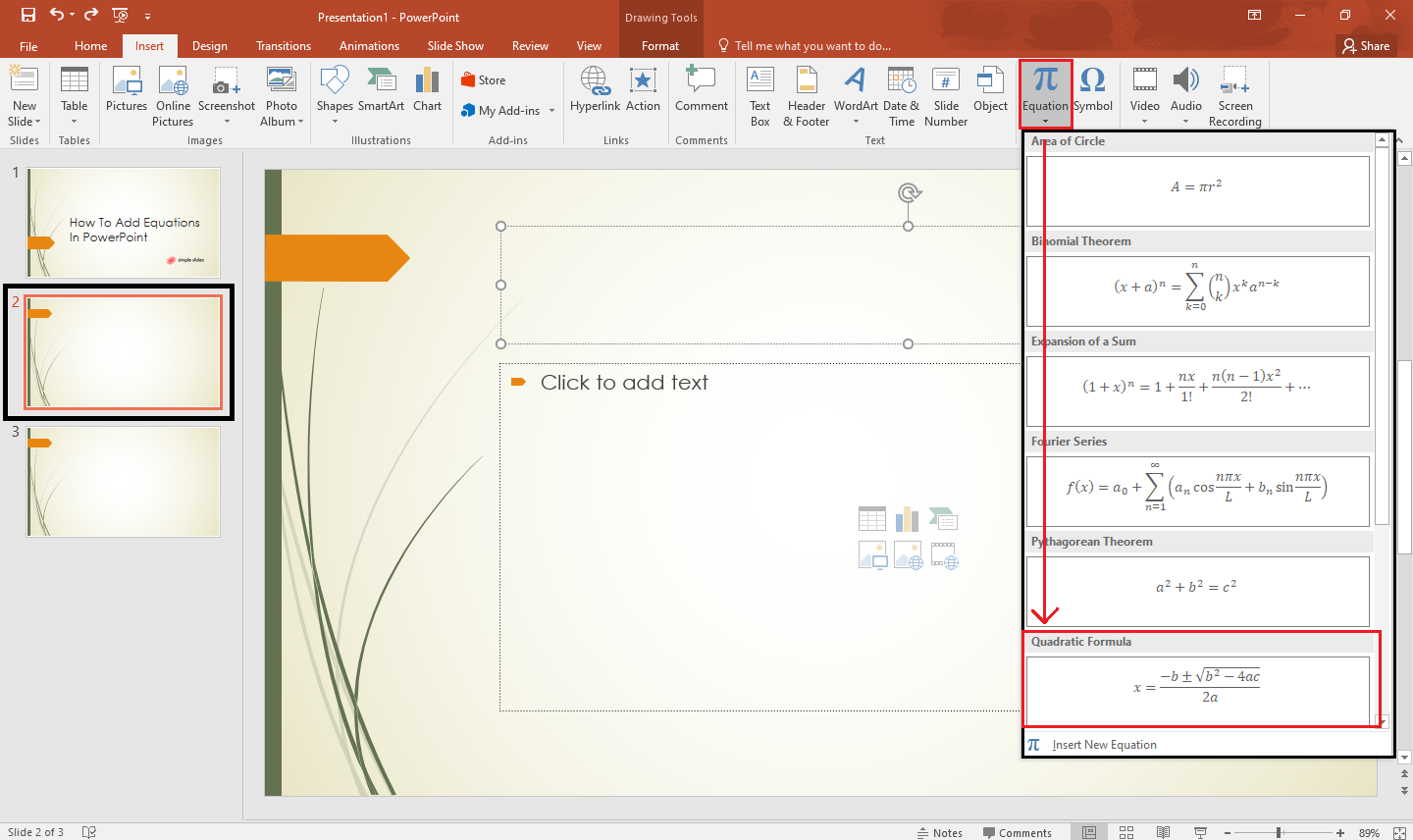4. Once you select an option for your equation, your equation will immediately insert into your PowerPoint slide.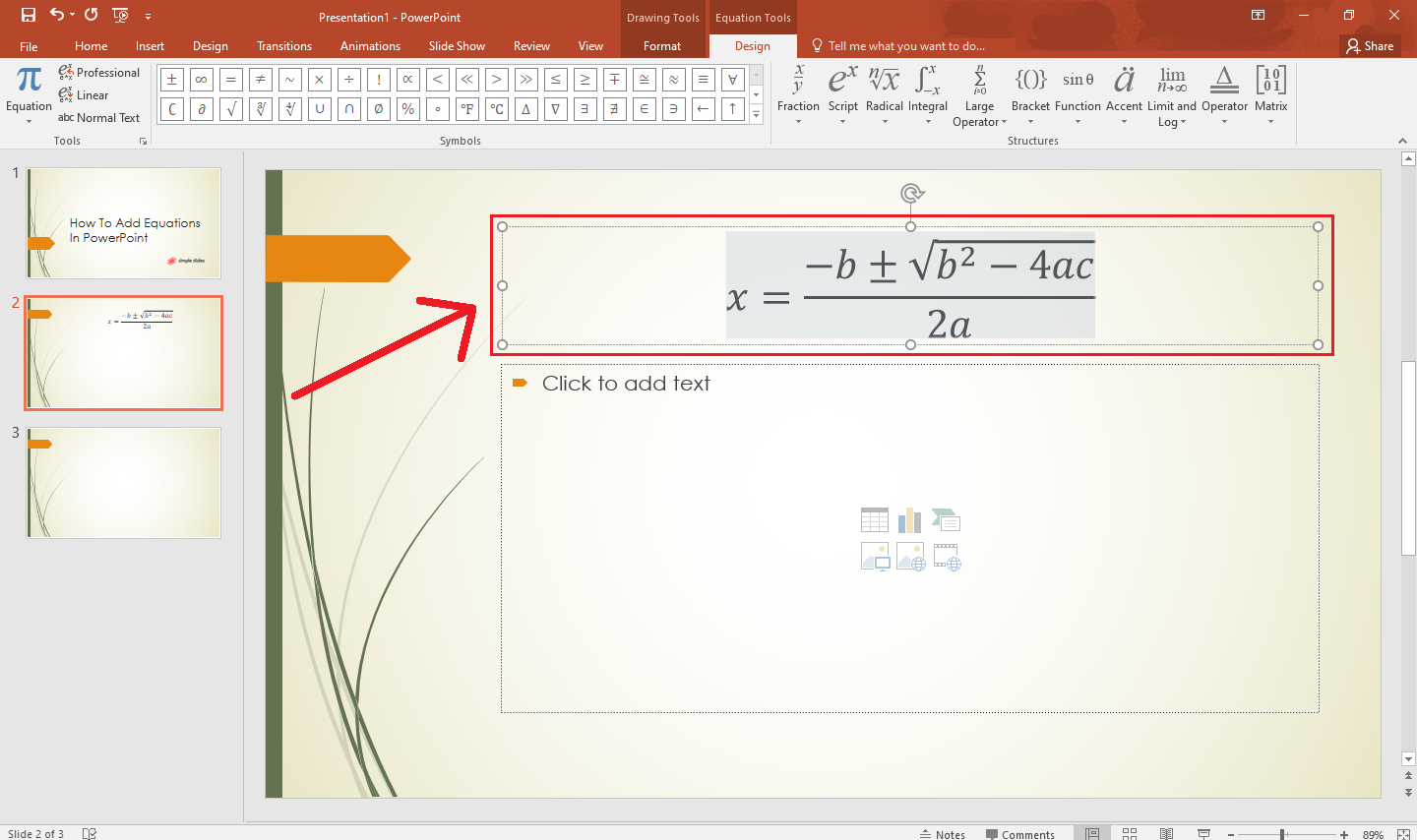If you want to customize your new equation on your presentation. in the "Drawing Tools" and "Equation Tools," select "Design" and select any function in the "Structures group" for your equation.

### Manually add a new equation.

If you want to input your entire equation to your presentation manually:

1. Select an existing slide on your presentation. Click the "Insert" tab and choose "Equation" under the "Symbol group."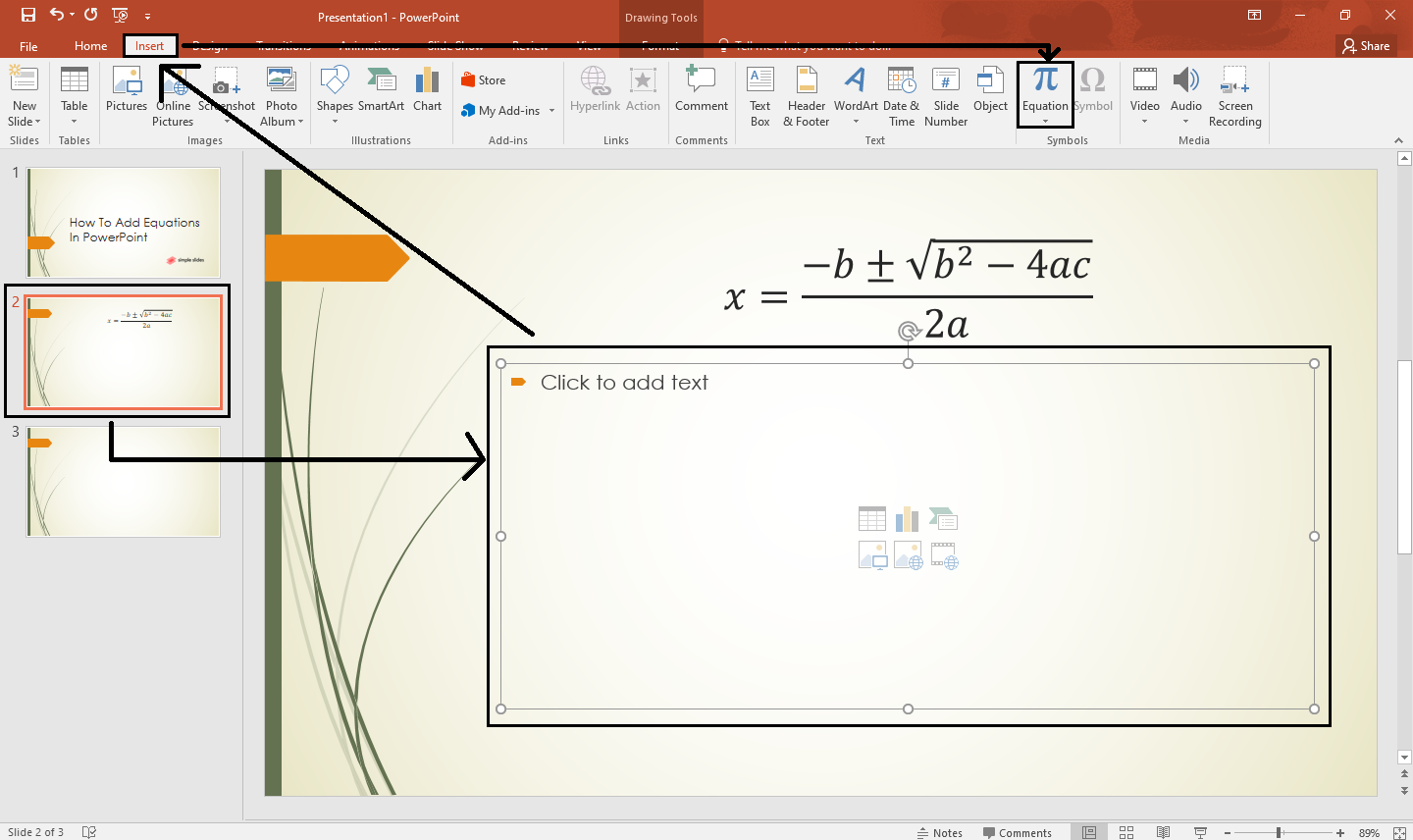2. In the drop-down menu, click "Insert New Equation."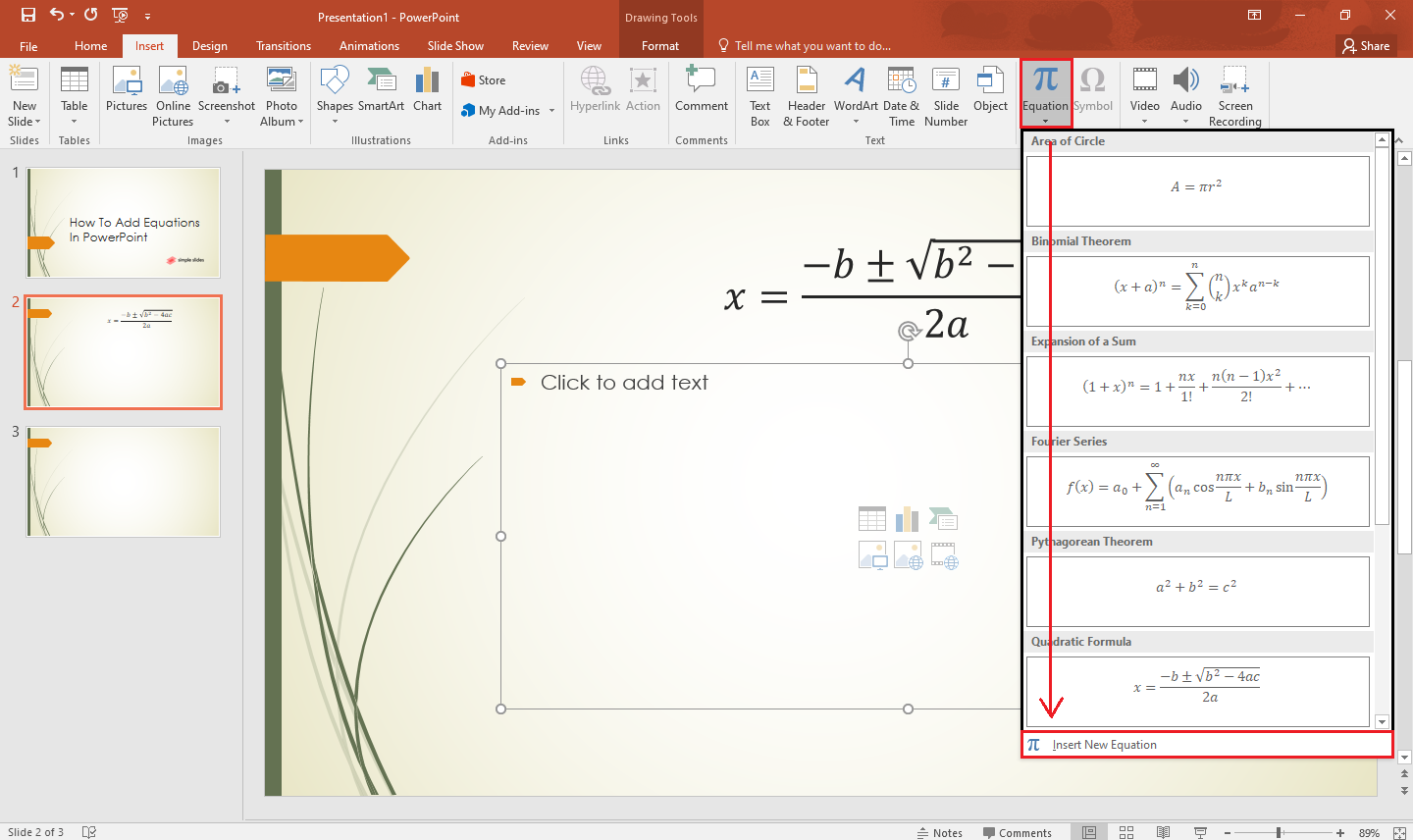3. Type your math equation using the options above in the "Structures" and the "Symbols" groups.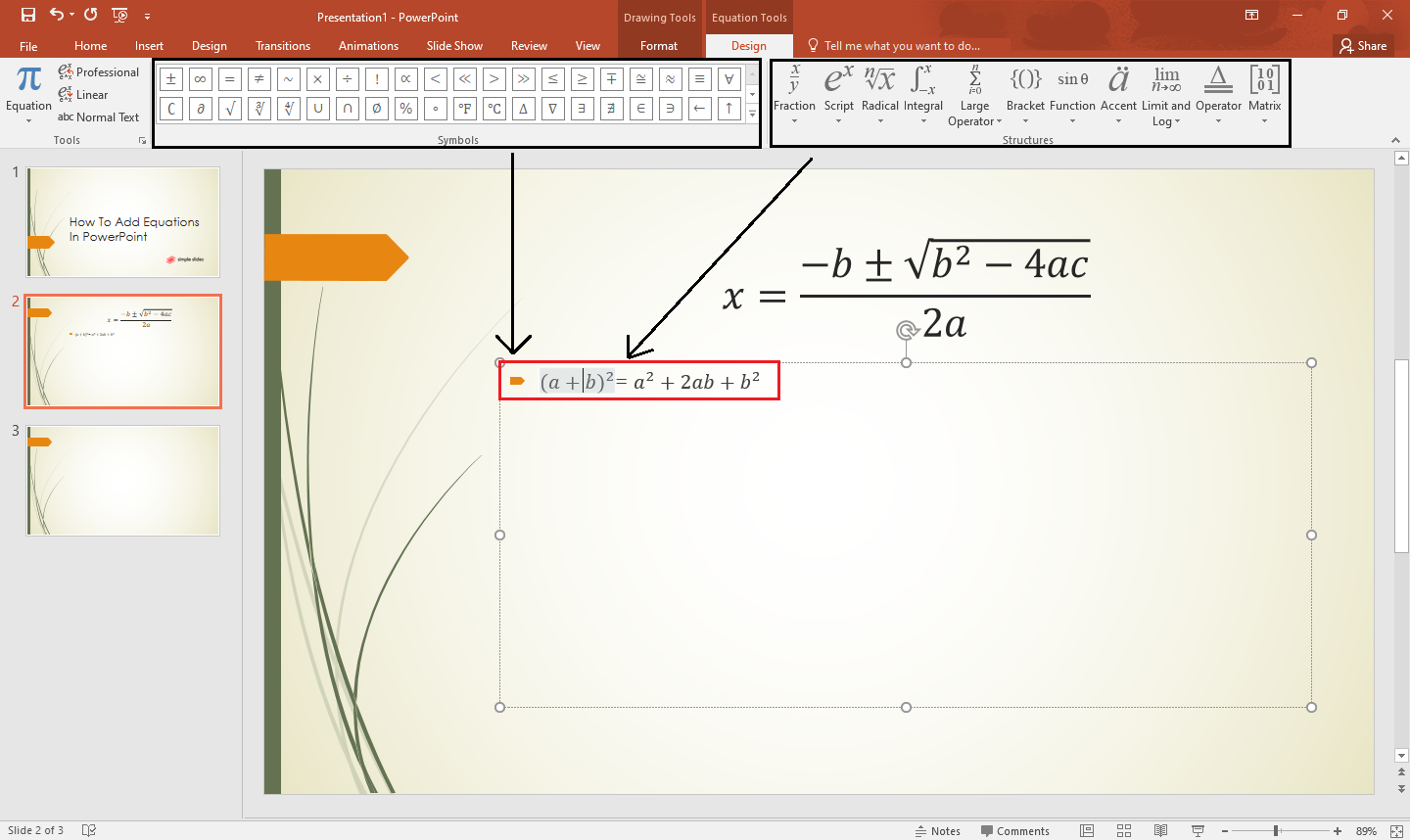4. After your new equation appears in the text box, press "Enter" once done.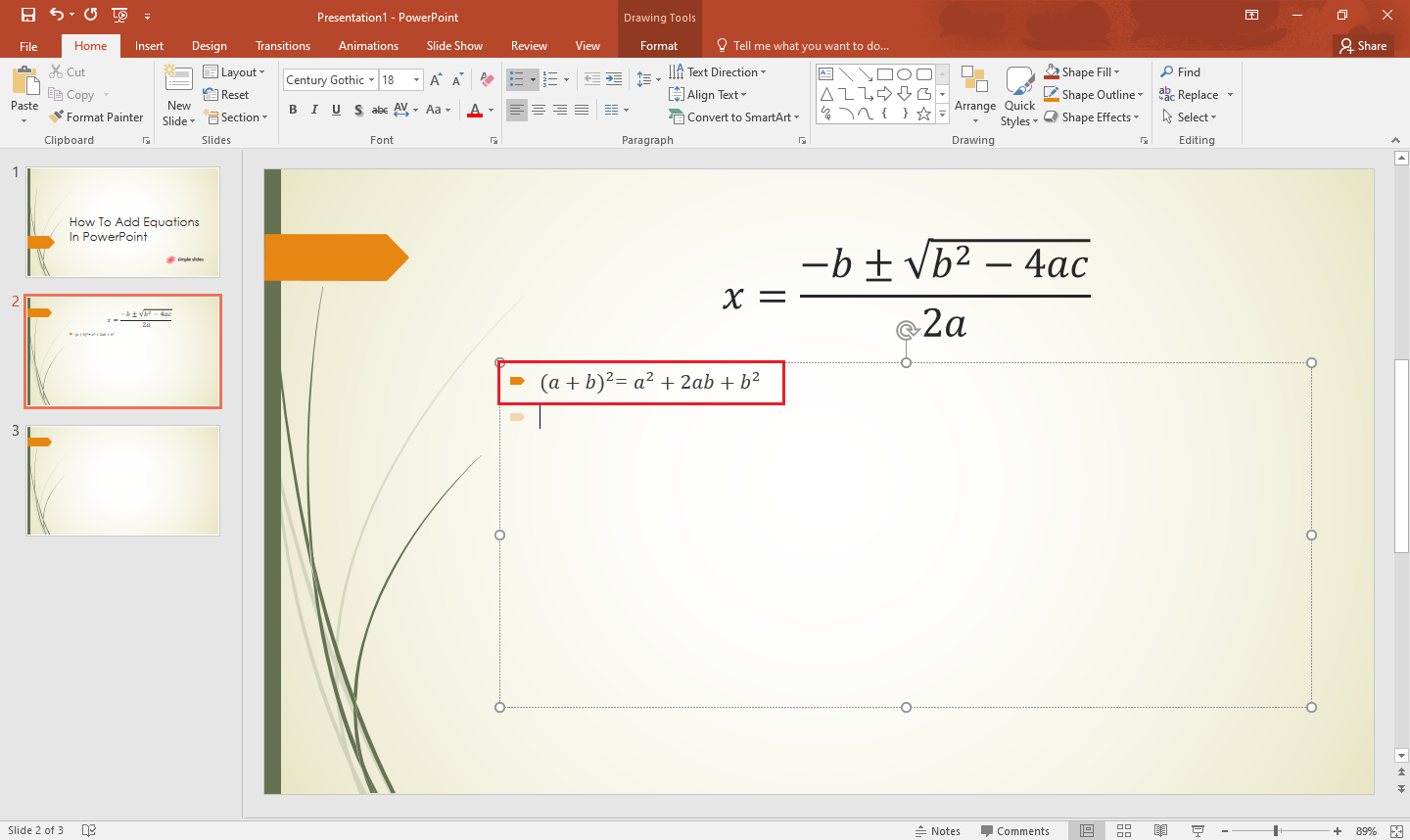### Customizing a Microsoft equation.

Suppose you want to change the font in writing your math equation in PowerPoint:

1. Highlight or select all the symbols you want to change.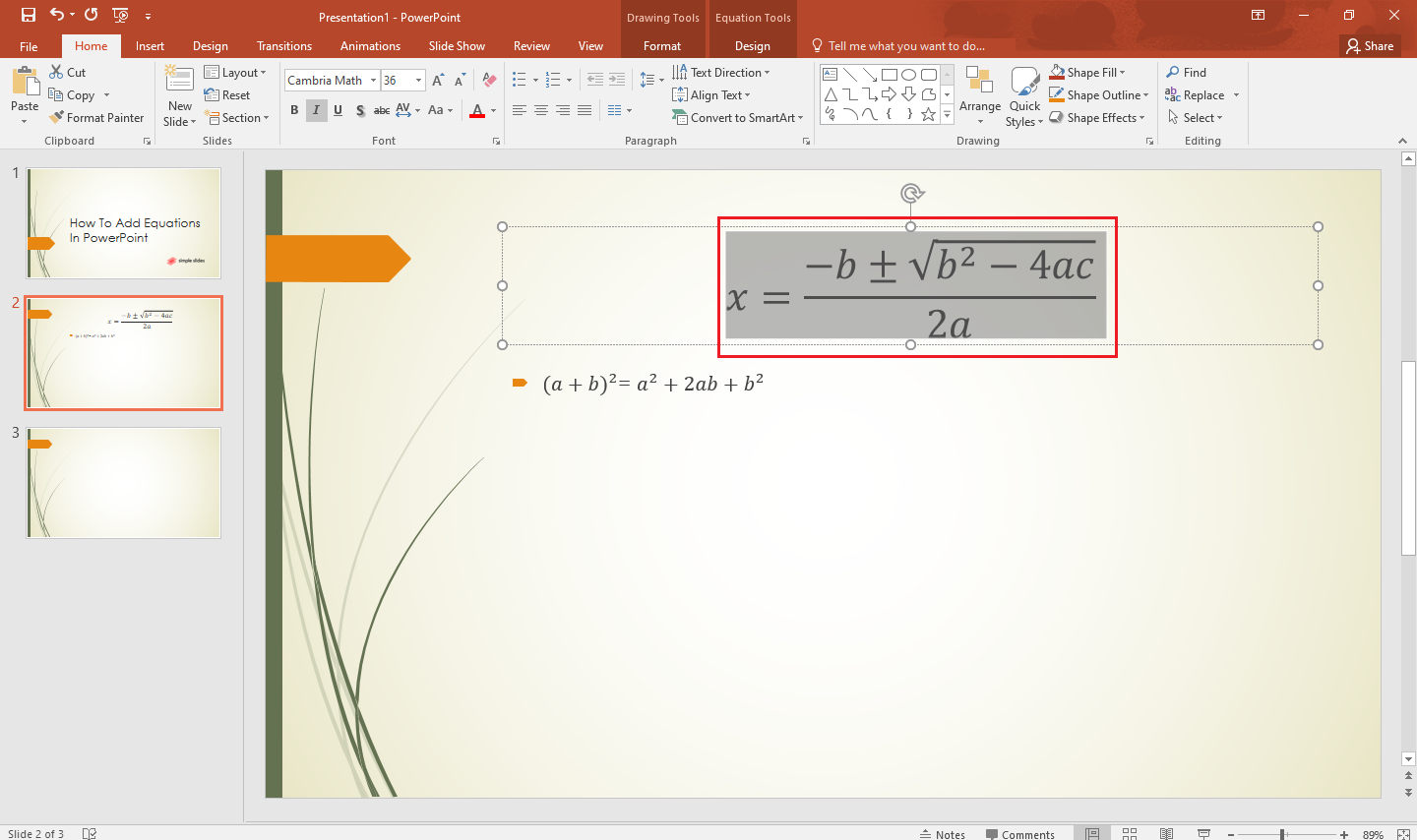2. Select "Design" under the "Equation Tools" and click the "Normal Text" option.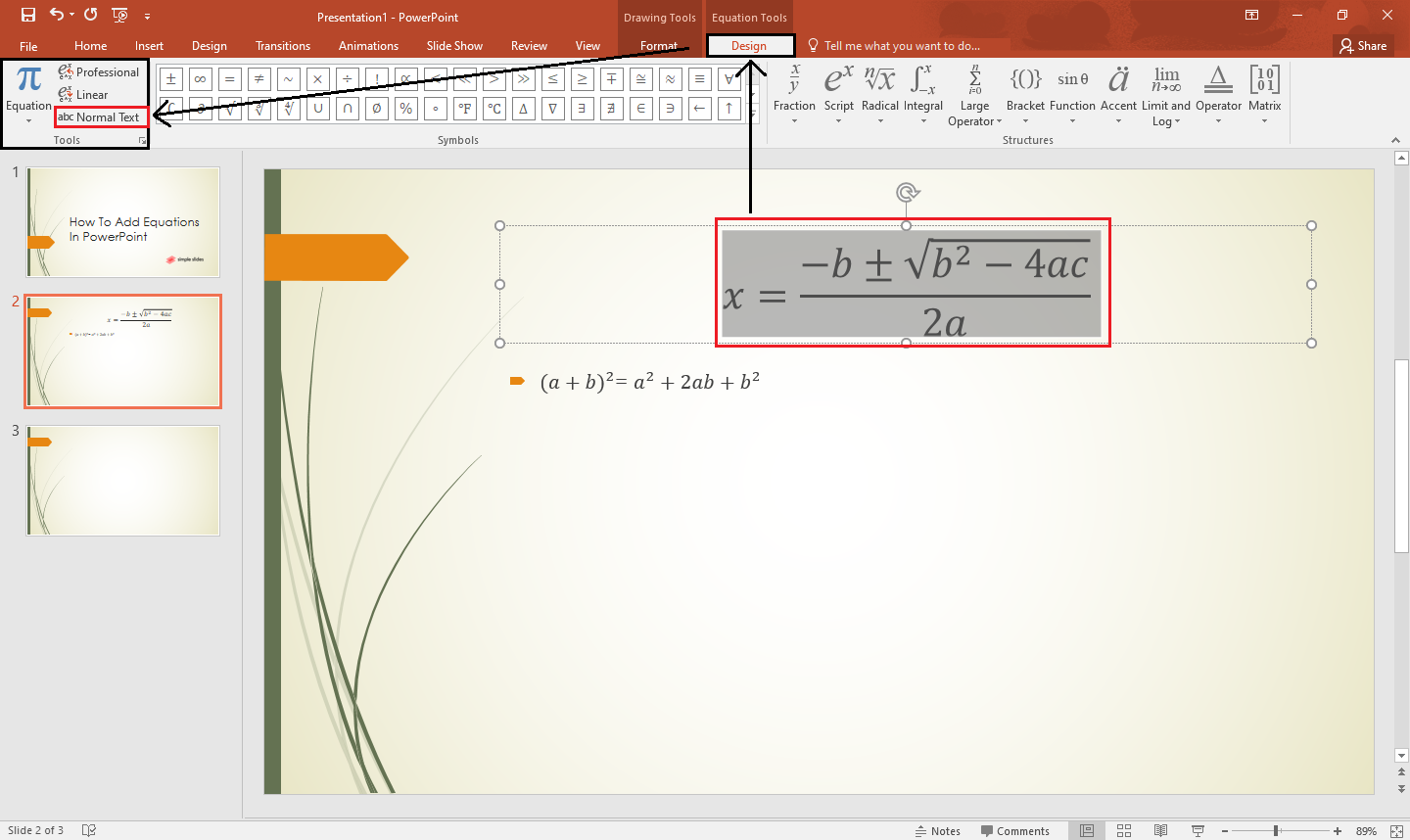3. After you click it, all your symbols will change their fonts.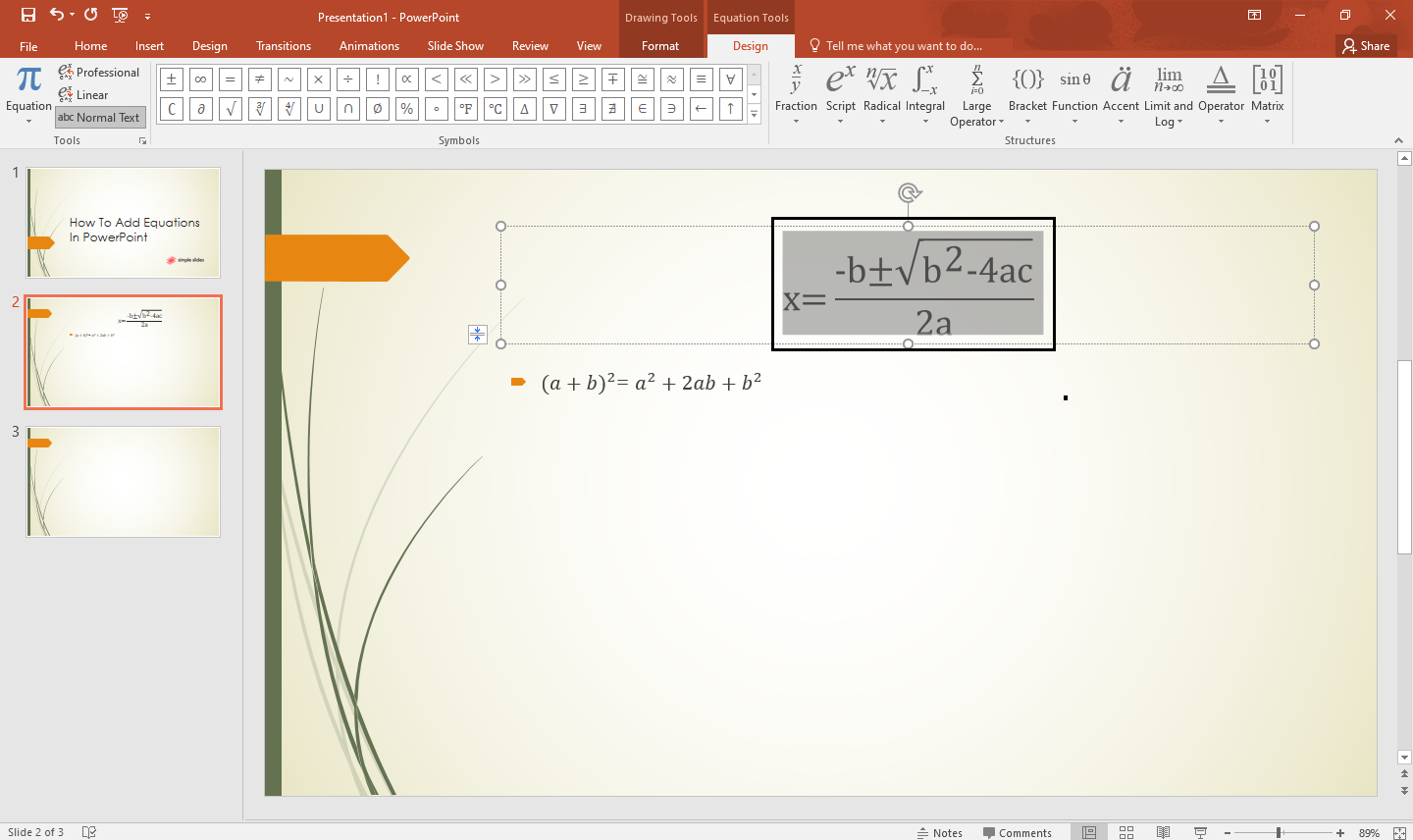## Final Thoughts on How to Add Equations to PowerPoint.

The two methods above are the most straightforward and quickest way to add new equations to your Microsoft PowerPoint.

But, If you want to make your PowerPoint presentation more fun and interactive for your audience, subscribe now, and access our latest templates and slides in Simple Slides.

## Frequently Asked Questions on How to Insert Equations in PowerPoint Presentations:

### What are the math equations available in PowerPoint presentations?

1. Area of the Circle

2. Binomial Theorem

3. Expansion of a Sum

4. Fourier Series

5. Pythagorean Theorem

7. Taylor Expansion

8. Trig Identity 1

9. Trig Identity 2

### Can I change the color format for my math equation?

Yes, you can change the size, type, and design of your math equation by; selecting the "equation," then going to the "Drawing Tool" & "Equation Tools" and selecting "Format." Navigate and click "Shape fill" to change the color of your math equation.

### What are the shortcut keys for "Equation" in PowerPoint presentations?

The shortcut key for "Equation" is Alt+N+E.## Related Articles:

How to Make Curved Arrows For PowerPoint: A Step-by-Step Guide

Charts For PowerPoint: Which One is Right for You?

How To Select PowerPoint Transitions in 4 Easy Steps

Get A Free Sample of Our Award-Winning PowerPoint Templates + University